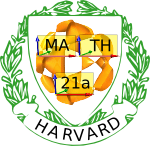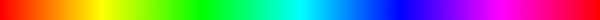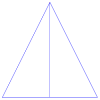Fall 2006

# Mathematics Math21a Fall 2006

## Multivariable Calculus

Office: SciCtr 434
Email: knill@math.harvard.edu
Harvard Mathematics

 Weekly checklists: "Somewhere, over the rainbow ..."Week1 Week2 Week3 Week4 Week5 Week6 Week7 Week8 Week9 Week10 Week11 Week12

## Homework

• Homework is due at the beginning of class. We do not accept electronic HW submission. If you do the homework with a word processor like LateX, print it out and submit the paper.
• No late homework accepted but will delete the least 3 scores for MWF sections and 2 for TTh sections. Reserve these 3 "Joker" cards for emergencies.
• Collaborations are welcome however you must turn in your own copy and list on it the names of your collaborators.
• Similarly, acknowledge the use of Mathematica. If you use Mathematica, nevertheless, write down all steps of your computation.
• If you can not turn in your homework because of a religious holiday like Yom Kipur, turn it in earlier or give it to a friend to turn it in.
• Homework scores from individual sections will be adjusted in the end because different graders grade each section.
• Please write legibly and indicate clearly, where a new problem starts.
• Try to keep the problems in order.
• Use words to explain your work, if necessary. Show your work.
• If unable to finish a problem, indicate where you are stuck. This will help us to give partial credit.
• Please infom about typos, misprints. We state here corrections to the in class distributed texts as well as to the posted solutions. Each hint gives you a bonus point. The texts posted here are corrected.
• (+Sol) means solutions have been posted. Solution folder (in case, solutions are not yet linked).

 ```Week 1: 1. Class: 11.2: 8,12,20,58,60 Due Wed (rsp Thu for TTh) 2. Class: 11.1: 34,36, 11.2: 76, 11.3: 20, 62 Due Fri (rsp Tue for TTh) 3. Class: 11.4: 8, 18, 38, 48, 54 Due Mon (rsp Tue for TTh) Solutions ```
 ```Week 2: 1. Class: 11.5: 16,20,40,64 (find distance),66 Due Wed (rsp Thu for TTh) 2. Class: 12.1: 26, 62 12.2: 12, 34, 50 Due Fri (rsp Tue for TTh) 3. Class: 12.3: 12,14,42, 56, 58 Due Wed (rsp Tue for TTh) Solutions ```
 ```Week 3: 1. Class: Happy Columbus day! 2. Class: 12.4: 10, 46, 13.1: 38, 46, 64 Due Fri (rsp Thu for TTh) 3. Class: 13.2: 16,22,26,28, 32 Due Mon (rsp Tue for TTh) Solutions ```
 ```Week 4: 1. Class: No Homework from Monday to Wednesday or Tuesday to Thursday 2. Class: 14.8: 4,10,19. There are additional custom problems: Problem A) invent a surface rho = f(phi,theta), simple enough to draw, but not in the book. Problem B) invent a surface r = g(theta,z), simple enough to draw but not appear in the book. You can use technology to play with but it is fine (probably even better), if you tackle the problem eventually without it. See FAQ. 3. Class: This problem is a custom problem set due Monday or Tuesday in the 5th week: A) Parametrize the upper half of the ellipsoid x2+y2+z2/4=1 in three different ways: as a graph r(u,v) = (u,v,f(u,v)) (Euclidean coordinates), as a surface of revolution r(theta,z) = (g(z) cos(theta),g(z) sin(theta),z) (cylindrical coordinates) and as a deformed sphere r(phi,theta) = (x(phi,theta), y(phi,theta), z(phi,theta) ) (spherical coordinates). B) The curve r(t) = (t1/2 cos(t), t1/2 sin(t), t) can be placed on a surface. Find a parametrization r(t,s) of this surface. C) Parametrize the surface which has distance 1 from the unit circle in the xy plane. This is a doughnut. Use two angles. D) Parametrize the paraboloid x2 + y2 = z in two different ways: as a graph or as a surface of revolution. E) Upload a picture for a gallery of marbles here Solutions ```
 ```Week 5: (We use frequently the notation fx(x,y) for the partial derivative of f(x,y) with respect to x). 1. Class 13.3: 10, 14. 13.4: 20, 32, 50 2. Class 13.4: 14, 52, 60, Problem A: verify that for any function g(x) of one variable, the function f(x,y) = g(x-y) + g(x+y) is a solution to the wave equation fxx(x,y) = fyy(x,y). Problem B: Cobb and Douglas found in 1928 empirically a formula P(L,K)= r La Kb for the total production P of an economic system as a function of the amount of labour L and the capital investment K. By fitting data, they got r=1.01, a=0.75, b=0.25. Verify that the function P(L,K) satisfies the partial differential equation L PL + K PK = P. 3. Class: 13.5: 2,6, 12, 44, 46 hint to 44 Solutions ``````Week 6: 1. Class 13.6: 2,8 13.7: 40, 42, Problem A: given g(x,y,z) = z-f(x,y). What is the relation between the gradient of g, a vector in space and the gradient of f, a vector in the plane? Describe it in general and explain it also with a simple example, where you can draw the level curves of f and the level surfaces of g. 2. Class 13.6: 20, 26, 48, 52, 56 3. Class 13.7: 12, 16, 30, 34, 38 (In problem 34, the "hypothenuse" is the base of the triangle.) Solutions ```
 ```Week 7: 1. Class: 13.8: 2, 18, 20, 30, 40 Problem 30: look for a minimum, not a maximum 2. Class: 13.9: 4,12,28,24,34 3. Class: no class: Veterans day ``` Solutions
 ```Week 8: 1. Class no homework (exam review) 2. Class: 14.1: 18, 44 14.2: 24,26 14.3: 26 Due: Friday, rsp Tuesday 3. Class: 14.5: 8,10, 30,54,60 Due: Monday, rsp Tuesday Solutions ```
 ```Week 9: 1. Class: 14.6: 8, 24,26, 36, Due Wednesday Nov 22 rsp Tuesday Nov 28 Problem 42 is deleted now 2. Class: 14.7: 2, 8, 16, 18, 20 Due Monday rsp Tuesday (after thanksgiving) 3. Class: no homework (thanksgiving) Solutions ```
 ```Week 10: 1. Class: 14.8 26,28,30, 32, 36 2. Class: 15.1: 6, 20, 26, 28, 48 (in 6 and 48 also draw some flow lines) 3. Class: 15.2: 2,6,20,38,40 Solutions and Solutions Addendum ```
 ```Week 11: 1. Class: 15.3: 10,12,22,34,40 2. Class: 15.4: 8,20,28,34,42 3. Class: 15.5: 6,10,12,26,28 Solutions ```
 ```Week 12: 1. Class: 15.6: 16 Problem A: compute the surface area of the surface r(u,v) = < v cos(u), v sin(u), v2 >, with u from 0 to Pi/2 and v from 0 to 1. 15.7: 28, 30 (compute only the flux integral), Problem B: compute the flux of F(x,y,z) = (0,0,z) through the torus parametrized as r(u,v) = < (2+cos(v)) cos(u), (2+cos(v)) sin(u), sin(v)>, where both u and v range from 0 to 2pi. Problem 15.7:32: delete =2 in the definition of the surface. The surface is the elliptic paraboloid z= 1-x2/4-y2, z>0 2. Class: 15.7: 14,16,18,32, Problem A: Find the flux of the curl of F(x,y,z) = <-y + x y z, sin(x y z), sin(cos(x y - z))> through the upper half of the sphere x2 + y2 + z2 =1, z > 0. The surface is oriented upwards. } 3. Class: 15.8: 2,10b,18b, Problem A: Use the divergence theorem to calculate the flux of F(x,y,z) = (x3,y3,z3) through the sphere x2+y2+z2=1. The sphere is oriented outwards. Problem B: What is the flux of the vector field F(x,y,z) = < sin(y) cos(z), sin(z) cos(x), sin(x) cos(y) > through the torus surface parametrized by r(u,v) = < (2+cos(v)) cos(u), (2+cos(v)) sin(u), sin(v) > where both u and v range from 0 to 2pi? Solutions ```